Anzeige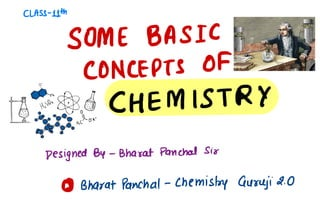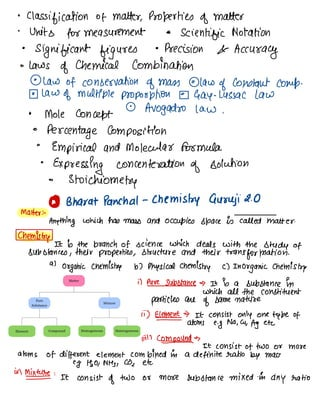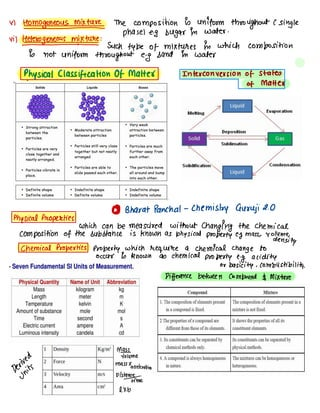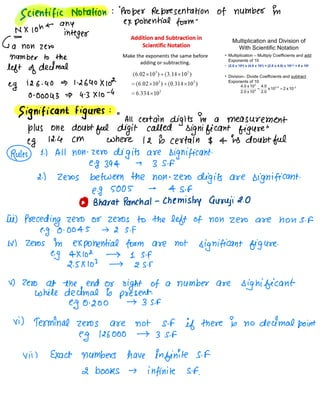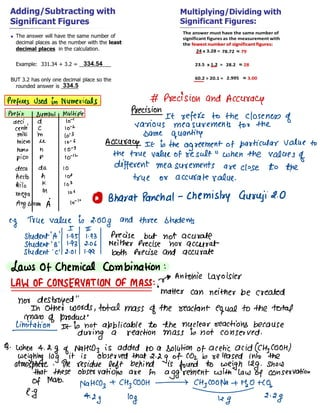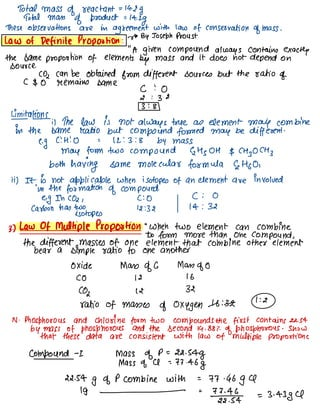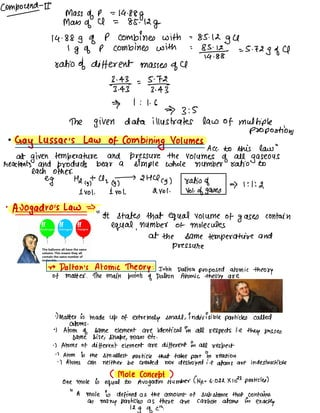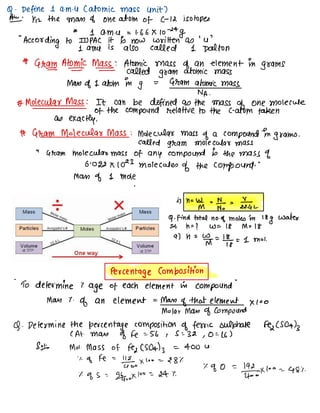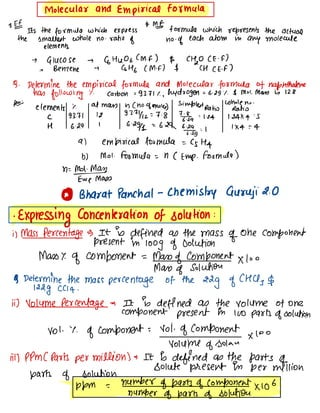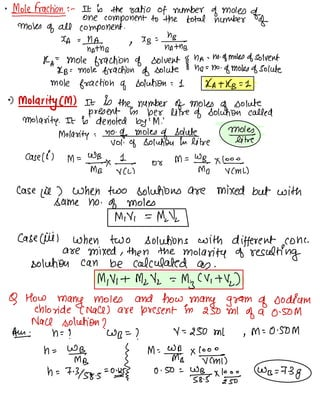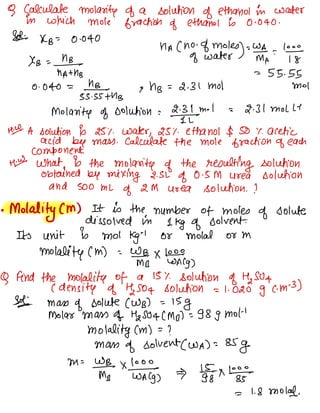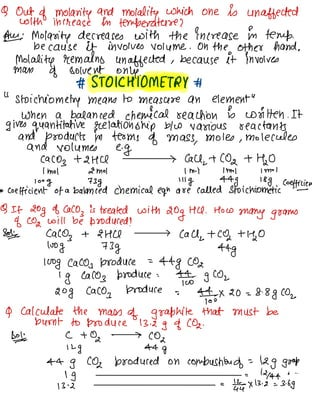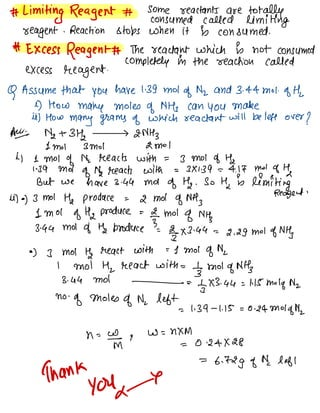1 von 13
Anzeige

### SBCOC By Bharat Panchal class 11.pdf

1. CLASS- 11th SOME BASIC CONCEPTS OF CHEMISTRY Designed By - Bharat Panchal Sir Do% Bharat Panchal - Chemistry Guruji 2.0
2. • Classification of matter. Properties of matter • Units for measurement • scientific Notation • significant figures • Precision & Accuracy • laws of chemical combination • law of conservation of mass • law of constant comp. • law of multiple proposition • Gay-Lussac law • Mole concept • Avogadro law . • Percentage composition • Empirical and Molecular formula • Expressing concentration of solution • stoichiometry matter . - BOB Bharat Panchal - Chemistry Guruji 2.0 Anything which has mass and occupies space is# alter. Chemistry It is the branch of science which deals with the study of substances , their properties, structure and their transformation. at organic chemistry b) Physical chemistry c) Inorganic chemistry it Pure substance It is a substance in which all the constituent particles are of same nature ii ) Element It consist only one teebe of - atoms e.g Na , ca, Ag etc iin (0M¥ It consist of two or more atoms of different element combined in a definite ratio by mass e.g Hao, Mtg, CO , etc. ith Mixture : It consist of two or more substance mixed in any ratio
3. v1 Homogeneousmixture The composition is uniform throughout C single phaseleg sugar in water . "it HÉmI¥ : such type of mixtures in which composition is not uniform throughout e.g sand in water • Physical Classification Of Matter Interconversion of states of Matter Gao Bharat Panchal - Chemistry Guruji 2.0 Physical Properties which can be measured without changing the chemical composition of the substance is known as physical property e.g mass, volume, density chemical Properties property which require a chemical change to occur is known as chemical property e. g acidity or basicity , combustibility. Difference between compound $Mixture Mads MH ed volume qeÑgjgs mass ✗ acceleration pisH¥me lxb 4. Scientific Notation : " Proper Representation of number in exponential form " N ✗ 10h ← any = integer C)a non zero number to the left of decimal e. g 126.40 1.2640×102 0-00043 4.3×10-4 Significant figures : " Au certain digits in a measurement plus one doubtful digit called significant figure " e.g 12.4 cm where 12 is certain$44s doubtful Rules 1-1 All non - zero digits are significant. e.g 394 → 3 Sif 2.) Zeros between the non - zero digits are significant. e.g 5005 → Asf Bo% Bharat Panchal - Chemistry Guruji 2.0 Iii) Preceding zero or zeros to the left of non zero are no ns.re.g 0.0045 → 2 5. F in zeros in exponential form are not significant figure. e.g 4×102 → 1 Sif 2. 5×103 → 2 Sir 4) Zero at the end or sight of a number are significant while decimal is present. e.g 0.200 → 3 5. F Yi ) Terminal zeros are not Sif if there is no decimal point e.g 126000 → 3 Sif Xiii Exact numbers have infinite s.FI books → infinite Sif.
5. Prefixes used in Numerical # Precision and Accuracy Precision Prefix Symbol Multiple deci d to -1 It refers to the closeness of anti c to - a various measurements too the Milli m lo -3 same quantity micro he 10-6 Accuracy It is the agreement of particular value to nano h 10-9 pico p so - in the true value of result " when the values of deca da lo different measurements are close to the hecto h 102 true or accurate value. kilo K 103 106 me " m µ . Go☒ Bharat Panchal - Chemistry Guruji 2.0 Angstrom A. e. g True value is 2.00g and three students I II student 'A ' 1.95 1.93 Precise but not accurate student ' B ' 1.93 2.06 Neither Precise nor accelerate student ' C' /2.01/1.99 both Precise and accurate Laws Of Chemical Combination : LAW OF CONSERVATION Of mass : v0 Antonie Lavoisier " matter can neither be created nor destroyed " In other words , total mass of the reactant equal to the total mass of product " limitation It is not applicable to the nuclear reactions because during a reaction mass is not conserved . Q. when 4.2g of Matteo} is added to a solution of acetic acid (CH , COOH) weighing log it is observed that 2.2g of CO2 is released into the atmosphere . The residue left behind is found to weigh 12g . Show that these observations are in aggrement with law of conservation of Mab. Matteo, + CHZCOOH -7 CHZCOONA -11%0+10 , e.g 4. 2g 10g kg 2.2g
6. Total mass of reactant = 14.2g Total mass of product = 14.2g These observations are in agreement with law of conservation of mass . Law of Definite Proportion : v0 BY Joseph Proust " A given compound always contains exactly the same proportion of elements by mass and it does not depend on source. CO2 can be obtained from different sources but the ratio of C $0 remains same c :O 2 : 32 3 : 8 Limitations ;) The law is not always true as element may combine in the same Matto but compound formed may be different . e. g C : H :O = 12 :3 :S by mass may form two compound ↳ Hs OH$ CH ] OCH } both having same molecular formula ↳ Hoo, ii) It is not applicable when isotopes of an element are involved in the formation of compound. eg In CO2 , c :O C : 0 carbon has two 12:32 14 : 32 isotopes 3.) Law Of Multiple Proportion " When two element can combine to form more than one compound , the different masses of one element that combine other element bear a simple ratio to one another oxide Mass of G Mass of 0 CO 12 16 (02 12 32 ratio of masses of oxygen -16 :32- 1 :& N - Phosphorous and chlorine form two compounds the first contains 22.54 by mass of phosphorous and the second 14.88% of phosphorous . Show that these data are consistent with law of multiple proportions compound - I mass of D= 22.54g Mass of A = 77.46g 22.54g of P combine with = 77.46g a 19 - = 72-2%-4 = 3.4384
7. Compound- II mass of P = 14.88g Man of Cl = 85.12g 14.88g of P combines with = 85.12 gu 1g of p combines with = 8Yj.gg#--5.72gofCl ratio of different masses of a 3. 43 = 5,7¥ 1:16 3 :S The given data illustrates law of multiple propositions • Gay Lussac 's Law of combining volumes Acc. to this law " at given temperature and pressure the volumes of all gaseous reactants and products bear a simple whole number ratio to each other. e. g Ha + Uz → 2116cg ) ratio of (g) (g) 11101. I v01. 21101 . v01 . of gases 1 : I :L • Avogadro's law " * states that equal volume of gases contain equal . Number ' of molecules at the same temperature and pressure ra Dalton 's Atomic Theory : John Dalton proposed atomic theory of matter . The main points of Dalton Atomic theory are e) Matter is made up of extremely small , indivisible particles called atoms . • 1 Atom of same element are identical in all respects i.e they posses same size, shape, mass etc . e) Atoms of different element are different in all respect • ' Atom is the smallest particle that take part in reaction • 7 Atoms can neither be created nor destroyed i. e atoms are indestructible Mole Concept One Mole is equal to Avogadro Number (Na = 6-022×1023 particles) " A mole is defined as the amount of substance that contains as many particles as these are carbon atoms in exactly 12g of c ' ?
8. g. Define 1 am - u C atomic mass unit) AI Hz the mass of one atom of C- 12 isotopes * I a. m.cl = 1.66 ✗ to -24g " According to IUPAC it is now written as ' u ' Lama is also called 1 Dalton * Gram Atomic Mass : Atomic mass of an element in grams = = = called gram atomic mass Man of 1 atom in g = Gram atomic mass NA . # Molecular Mass : It can be defined as the mass of one molecule of the compound relative to the c-atom taken as exactly. # Gram Molecular Mass : Molecular mass of a compound in grams . called gram molecular mass " 6ham molecular mass of any compound is the mass of G. 022 ✗ 1023 molecules of the compound. " Man of 1 Mole E- is n=W_m = ^¥ = ¥-4b 9. Find total no - of moles in 18g water Sol. n = ? w= 18 M= 18 9) h = % = 187g = I Mot. Percentage composition • To determine % age of each element in compound " Man % of an element = Man of that element ✗ too Molar man of compound G. Determine the percentage composition of ferric sulphate redcoats CAT. Man of Fe = 56 , 5=32 , 0=16 ) 8¥ Moi . Mass of Fey (Soa) } = 400 he i. of Fe = III.• ✗ too = 28% % of 5 = 96-4. • ✗ too = 24% % 90 = 1%-5×1- • = 48%
9. Molecular and Empirical formula * E¥ Its the formula which express * M¥ formula which represents the actual the smallest whole no . ratio of no . of each atom in any molecule elements → Glucose → ↳ His 06 ( Mrf ) $CHAO CE - f) → Benzene → ↳ Hf ( Mrf)$ CH C E. f) 9. Determine the empirical formula and Molecular formula of naphthalene has following % carbon = 93.71% , hydrogen = 6.29% $Mol. Man is 128 whole no . MY elements T .at man hcnocfomoled simplest ratio ratio c 93.71 12 93^-412=7.8 76.8-29 = 1.24 1.24×4--5 H G. 29 I 6-2911 = 629 6 ?÷g :| 1×4--4 a) empirical formula = G- Hq b) Mol . Formula = n C Emp. formula ) n=m÷?ñ→ Do% Bharat Panchal - Chemistry Guruji 2.0 • Expressing concentration of solution : i , mass percentage It is defined as the mass of one component present in 100g of solution Massy. of component = Mass of component ✗ too Man of solution 9 Determine the mass percentage of the 22g of CHU ]$ 122g CCI 4 . ii) volume percentage as It is defined as the volume of one component present in in parts of solution V01 . % of component : V01 . of component Volume of Solan ✗ 100 Iii) PPMC Parts per million ) It is defined as the parts of solute present in per million parts of solution ppm = number of parts of component ✗ 106 number of parts of solution
10. • Malefactions: - It is the ratio of number of moles of one component to the total number of moles of all component . XA =NA_ hath☐ 1 "B =n?n%-n, RA= Mole fraction of solvent § ha - - no - of moles of solvent KB : mole fraction of solute hrs = no of moles of solute mole fraction of solution = 1 RATKB =L • ) Molarity (M) It is the number of moles of solute present in per litre of solution called molarity. It is denoted by 'M ! Molarity = no - of moles of Solute v01 . of solution in like Mef¥ case lil M=wmB_☐✗,÷y or M=%☐×Y÷↳ case lie ) when two solutions are mixed but with same no . of moles MY = Mdk case ciiil when two solutions with different cone- are mixed , then the molarity of resulting solution can be calculated as . Milli -1 Milk = My (4+11) Q How many moles and how many gram of sodium chloride C NaCl ) are present in 250 MI of a 0.50M Nacl solution ? AE n= ? WB = ? 4--250 MI , M - -0.50M n= WI , { M :#☐ ✗ ' ÷÷, h = 7-3158.5=011-5 0.50 = wgg-s.tl;÷ Wrs = -73g
11. 9 Calculate molarity of a solution of ethanol in water in which mole fraction of ethanol is 0-040 . Sdi KB = 0.040 nA ( no - of moles wa of water )=_ma : ' ÷ ✗B = MBNA + hrs = 55.55 0.040 = hB_ Mol 55.55 + hrs 9 NB = 2.31 MOI molarity of solution = ¥4m - I = 2.31 Moll-1 Hit A solution is 25% water, 25% ethanol \$50 % acetic acid by mass . Calculate the mole fraction of each component. Hid what is the molarity of the resulting solution obtained by mixing 2.5L of 0.5M Urea solution and 500 mL of 2M uoea solution . ? • Molality ( m) It is the number of moles of solute dissolved in 1kg of solvent. Its unit is moi kg " or molal or m molality ( m) = wmB_☐✗%¥g) Q And the molality of a 15% Solution of Hasan C density of Hasoq solution = 1.020 g c. m - 3) Sofa mass of solute ( WB) = 15g Molar mass of 112504 ( Mg) = 98g Mot ' molality (m ) = ? Mass of solvent( WA) = 85g m=wm÷×"w%-g, ¥g✗'%É- = 1.8 Mold.
12. Q Out of molarity and molality which one is unaffected with increase in temperature ? Aus Molarity decreases with the increase in temp. because it involves volume . On the other hand . Molality remains unaffected , because it involves man of solvent only. # STOICHIOMETRY # " stoichiometry means to measure an element " when a balanced chemical reaction is written . It gives quantitative relationship b/w various reactants and products in terms of mass , moles , molecules and volumes e.g Caco } -12 HCl 7 Call, -1 CO2 + Hao 1m01 2m01 1 Mol 1m01 Im-1 100g 73g 111g 44g 188 Coefficient * coefficient of a balanced chemical eqn are called stoichiometric - Q If 20g of Caco , is treated with 20g HU . How many grams of CO2 will be produced ? Sots Caco , + 2116 > call, + CO2 -1110 loog 73g 44g 100g Caco, produce = -44g CO2 1g Caco, produce = 9% g coz 20g Caco , produce = 4,4-0×20=8-8g coz 9 Calculate the mass of graphite that must be burnt to produce 13.2g of CO2. 80¥ C +02 → cog 12g 44g -44g CO2 produced on combustion of = 12g goop 1g = 12144 " " 13^2 = 1-4×13.2 =3.bg
13. # Limiting Reagent # some reactants are totally consumed called limiting seeagent . Reaction stops when it is consumed . * Excess Reagent# The reactant which is not consumed completely in the reaction called excess reagent. Q Assume that you have 139m01 of Nz and 3. 44m01. of the e) How many moles of NH , can you make it) How many grams of which reactant will be left over ? Aye Ms -13112 > 2MHz 1m01 3m01 2m01 it 1m01 of N, reacts with = 3 Mol do Ha " 39 Mol of Ng reacts with = 3×139--4.17 mad of Ha But we have 3.44 moi of Ha . So this limiting it)•) 3m01 Ha produce = 2 Mol of NH , Reagent . 1m01 of H2 produce = f- Mol of MHz 3.44 MOI of Ha Produce ' = 2-3×3.44 = 2.29 Mol of Mtf • ) ] Mot tf react with =L Mol of N , I Mot Hz react with = tgrnol of Nff 3. 44 MOI - = tg ✗3.44 = 1.15 Molq Nz no - of Moles of Nc left = 1. zq -1.15=0.24 Mot dona h = Im g w = NXM = 0.24×28 = 6.72g of Nc left Thank yoke
Anzeige